# The Chicken or the Beef? Why Everyone Loves Neural Networks

April 15, 2019 in Python Articles

Written by Ra Inta

You may have heard of the powerful, almost magical, tool of Deep Learning, or even may know something of Machine Learning using an Artificial Neural Network. What powers this magic? Here we try to give a simple illustration of what the fuss is all about, and even show you how to cast your own Artificial Neural Network spells — using the popular Python programming language along the way.

Let's say you have an important decision to make. You are hungry and wish to be sated. However, you are on a long airplane flight, so your options — as usual — are either 'chicken' or 'beef'. Your target variable is 'satisfaction,' which will not be achieved if you choose neither, or both, options. You must make this decision while you have the flight attendant's attention. Finally, the attendant is new and very busy, and may not recall your first choice, so you will have to be explicit about what you do not wish, as well as what you do (or perhaps they ran out of one option and another attendant has to provide this option later, without information on your alternate meal choice).

This scenario is obviously contrived. Yet it doesn't take much to generalize this to more practical situations. So how would we go about modeling this decision? Our common go-to tool might be a linear regression, perhaps the most widely used statistical model. We have two input variables — our meal options — chicken (x1) and beef (x2). We wish to optimize our satisfaction function, which we'll call f(x). Recall the equation for a straight line is y = f(x) = mx +b, where m is the slope of the line, and b is where the line intersects the y-axis (the intercept). This generalizes to two x's as: f (x) = w1x1 + w2x2 + b, where the two wi are the weights (slopes) of the input variables, xi (the 'weight' terminology will become clearer later).  The usual procedure would be, because nothing is perfect in the real world of messy data, to approximate this linear function by minimizing some error function (such as least squares) from the observations (here just the possible values of the input variables).

A keen observer may already note the fatal flaw of this model. The meal choice depends entirely on the interaction between the two input variables, 'chicken' or 'beef'. If x1 is maximal (say 1), x2 would have to be minimal (0), and vice-versa (Figure 1).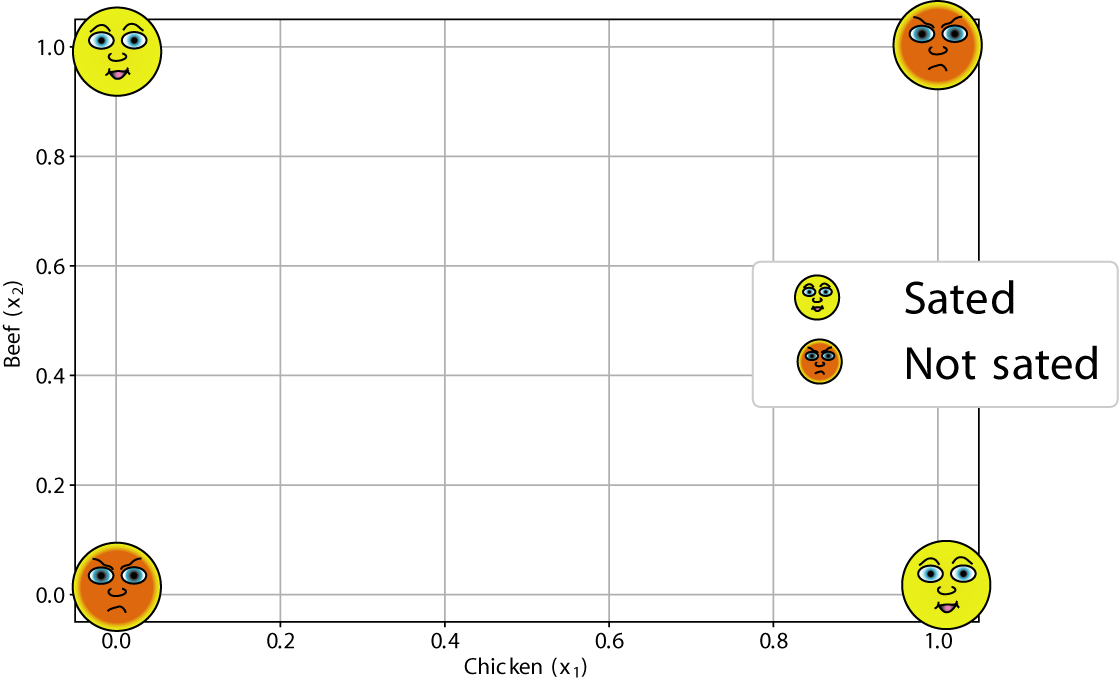Figure 1: The usual choice between meals on a long airplane flight: 'chicken' or 'beef'. You will be satisfied only if you choose exactly one option — you wish to suffer neither craving nor crapulence! In the field of Operations Research, this graphical depiction of choices to be made between various options or parameters is known as a decision plane. So this is a decision plane for meals... on a plane!

But we can't just change the coefficients from a regression at will! Once we have settled upon a model, the wi are set. In other words, there is no single line that separates the Chicken-Beef (x1 - x2) plane to define a distinct decision boundary between the two classes, 'sated' and 'not sated'. However, we may do this with two lines (and logical comparisons):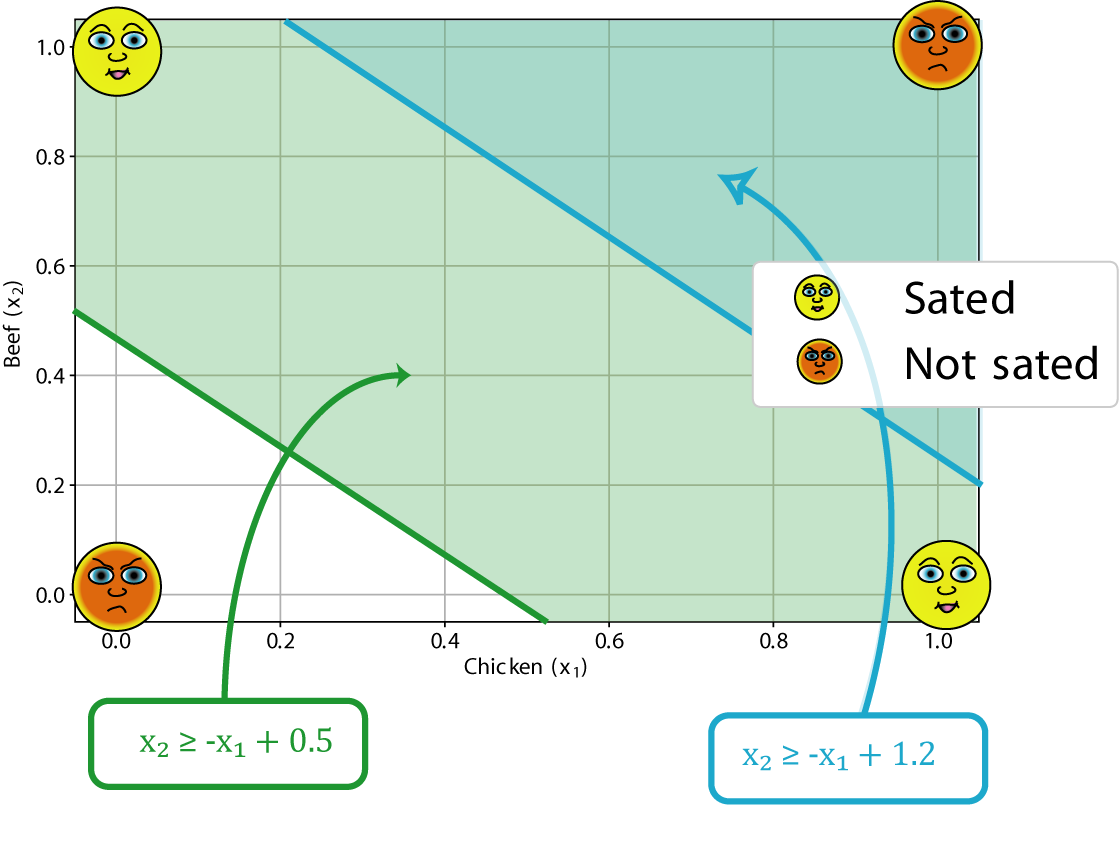Figure 2: A perfect classifier for our satisfaction function is obtained by using two linear regions on the Chicken-Beef decision plane.

The lower (green) line marks a boundary between the (0, 0) point and the other three, containing all but the origin point. The upper (blue) line defines a region that contains only the (1, 1) point.

In other words, the colored regions are defined by:

x2 ≥ -x1 + 0.5

x2 ≥ -x1 + 1.2

In other words: test the sum of x1 and x2. If it is greater than 0.5, the first equation is true. If greater than 1.2, the second is also true. Note that the actual numbers here don't matter: as long as we can separate the 'sated' and 'not sated' points, we will formulate our satisfaction. I arbitrarily chose equal slopes (unity) for both lines.

The simplest function to return 'true' or 'false' depending on a fixed threshold, is the Heaviside step-function, H(x). Despite sounding technical, it has the following simple properties: H(x) = 1 for x > 0 and H(x)=0 for x =0 (See Figure 5).

So our equation may be re-cast:

x1 + x2 ≥ 0.5 → H(x1 + x2 - 0.5)

x1 + x2 ≥ 1.2 → H(x1 + x2 - 1.2)

Finally, we then want these two lines to 'interact' with each other. We will only be satisfied if the first equation is true, and the second is false. A schematic allowing this interaction between the two lines may look like: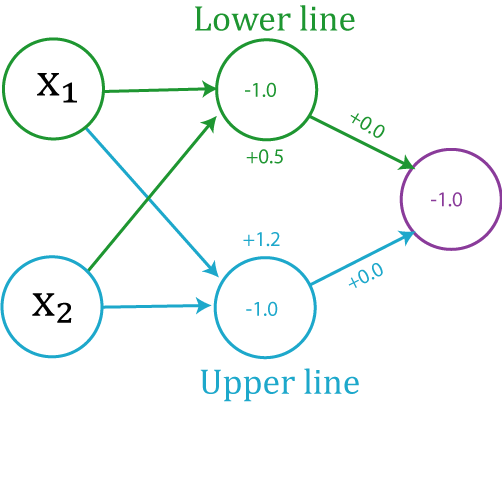Figure 3: Alternative representation of how to evaluate our satisfaction function. The numbers in the middle and top of the circles represent the weight (slope) and bias (intercept) values respectively. This is close to the conventional way artificial neural network architectures are represented

Where the last calculation is a simple logical comparison (and) of the regions.

This example, which is a surreptitiously colorful construction of the logical XOR function,  illustrates the essential idea behind the most fundamental neural network: the perceptron. We have just seen that, while a trusty linear regression failed to model our meal satisfaction function, a collection of simple units, that can easily interact and compare with each other, performed the task quite nicely.

## Artificial Neural Networks (ANNs)

Artificial Neural Networks (ANNs; often further abbreviated to NNs) are inspired directly from our understanding of brain neurophysiology. An individual neuron is the basic cell unit of our complex central nervous system. Each neuron takes inputs, in the form of electrical signals, and performs several simple transforms on these inputs, resulting in a simple output. These outputs are in turn fed as inputs to other neurons.

Artificial neurons are simplified analogs of these biological units, taking in a limited number of signals, performing simple operations on them, before emitting a limited number of output signals. The astounding computational capabilities of this class of algorithm arise from the networks built up using these simple units.

Extending this biological analogy, an ANN is composed of neurons (nodes) and layers. Each node performs the atomic operations of the network, defined by simple activation functions. Groups of nodes may form a layer, a distinct structure representing a stage of the network. Each layer acts like a filter, or function. At least two layers are defined: the input layer and the output layer.

In addition, there may be one or more layer that is neither an input nor an output; these are referred to as hidden layers: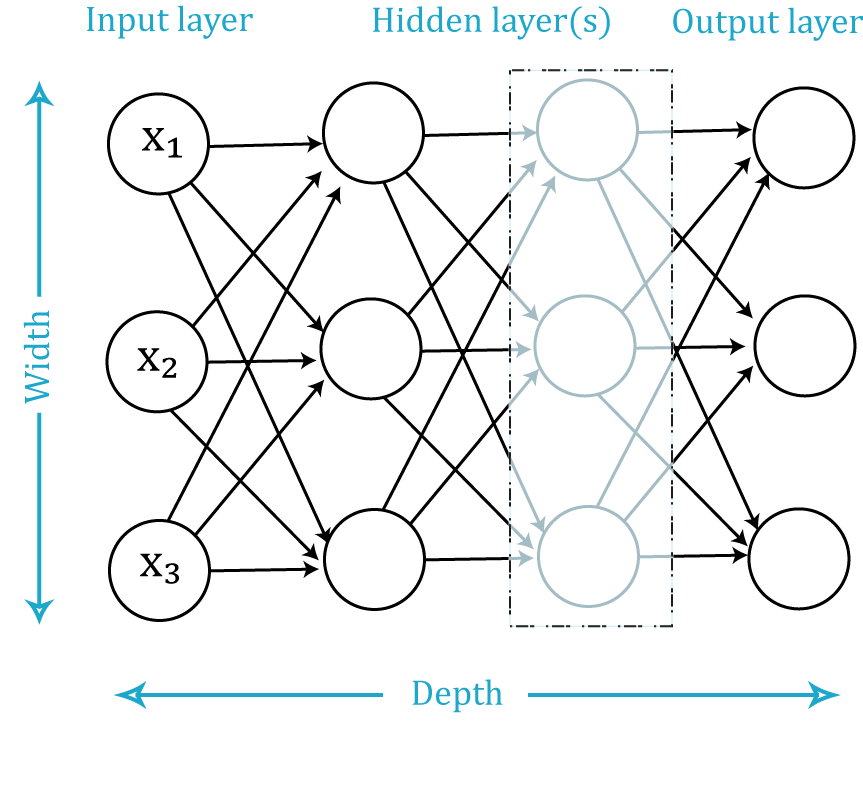Figure 4: Anatomy of an Artificial Neural Network. Each circle represents a neuron, or node. Most architectures tend to form distinct vertical groups, or layers. Neurons in each layer contribute to the width, while more layers contribute to the depth of the model.

The purpose of ANNs is to approximate any arbitrary (continuous) function, say, f'(x). Each layer can be thought of as a successive function fi() acting on the previous layers. The particular composition of layers and nodes of a neural network is known as the network architecture.

In this framework, for the chicken-or-beef calculation example, each linear comparison was performed within a node (neuron), after being fed inputs x1 and x2. The outputs were fed into the final, output, node for comparison. This architecture is known as a Multi-Layer Perceptron (MLP). It is a particular type of feed-forward network, because there are no layers that make use of feedback.

The activation function we chose (fairly organically!) was the Heaviside step function, H(x).

The purpose of an activation function is to polarize the network (i.e. provide directionality), as well as condition the signals propagated throughout (very often regulated to have a limited output range). The most common activation functions are:

Heaviside (Perceptron): H(z) = 1 for z > 0; 0 for z ≤ 0

Sigmoid (logistic): σ(z) = 1/(1 + exp(-z))

ReLU (Rectified Linear Unit): σ(z) = max(0, z)

Softmax: σ(z)j= exp(zj) Σ Kk exp(zk)

Their response functions look like the following: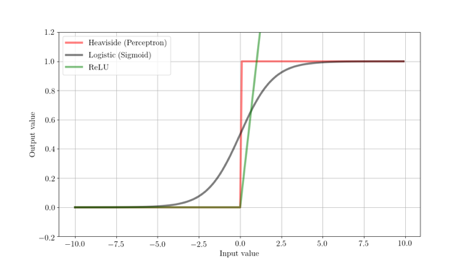Figure 5: Response functions for three of the most common activations functions used for artificial neurons. The Heaviside function (red curve) was the activation function we used for the chicken-or-beef example.

The sigmoid (or logistic) activation function is a smoothed version of the step function, so has nicer analytic properties than the step function (we'll see why this is important soon). However, it can be somewhat computationally expensive for large numbers of nodes and layers. The ReLU (Rectified Linear Unit) is a simpler function. Although, being piece-wise linear, it is still technically non-linear, it provides network polarity while retaining many properties of linearity which make these nice for approximating functions. ReLU-based neurons are much 'faster' to train because of their computational simplicity.

The softmax activation function is an ensemble function, often used for an aggregation step, so is great for neurons in an output layer. It also has nice analytic properties, regulating the output based on the ensemble mean. This often favors a 'winner-takes-all' condition.

## Constructing a Conceptual Artificial Neural Network

As mentioned above, ANNs provide a powerful way to arbitrarily approximate a continuous function. In the next article, we will use the concept to approximate our chicken-or-beef satisfaction function, showing this property of 'universal approximation' in action.

However, when we do, we are going to be lazy about it. Instead of going to all the trouble of measuring the appropriate weights and biases for each neuron, by graphing the decision plane and clustering the points by hand, we'll take a different approach. In fact, the manual calculations we did for this exercise are only feasible for very simple, linearly separable, problems, such as our contrived example.

In this first part, we saw how we could construct a mathematical framework to encode our satisfaction with meal choices on a plane. We took care and time to hand-craft the weights for the neurons ourselves. In Part 2, we will take a different track and see how easy it is to encode this simple problem using the Python libraries TensorFlow and Keras.

Accelebrate offers a wide array of Python training courses, including Introduction to Python, Advanced Python, Comprehensive Data Science with Python, Python for Data Analysis, and more.

 Of course, this scenario does not apply to business or first-class passengers. This would require a minor generalization of the model presented here. Plus additional funds... for empirical data collection purposes.

 Substitute for your favorite vegetarian, breatharian, vegan or other dietary preferences.

 Oliver Heaviside was one of the most famous English electrical/telecommunication engineers of the late 19th century. He was the first to propose the existence of the ionosphere, correctly calculated the geological age of the Earth, and transformed the mathematical frameworks for transmission of electromagnetic radiation and telecommunications to that which we still use to this day. He had a penchant for granite furniture and regularly painted his nails "a glistening cherry pink".

 The XOR, or 'exclusive or,' function outputs true only when the inputs differ — exactly our satisfaction function!

 The electronic Perceptron — a name that would fit perfectly in the Twilight Zone, but was invented two years before the first episode of that show — was an invention of Frank Rosenblatt, a psychologist, neurobiologist and electrical engineer. It visually recognized simple geometric objects, sparking huge interest in artificial intelligence throughout the late 50s and through the 60s. He also built an observatory at his home, thereby joining the Search for Extra-terrestrial Intelligence (SETI) effort.

Written by Ra IntaRa is originally from New Zealand, has a PhD in physics, is a data scientist, and has taught for Accelebrate in the US and in Africa. His specialties are R Programming and Python.